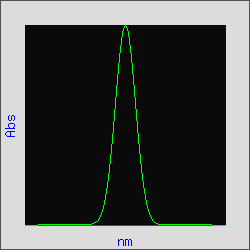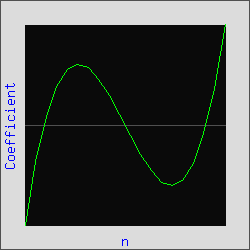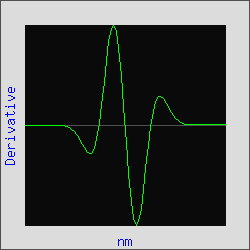Parameters
Polynomial(M) Derivative(d):
Filter length(f): Scale:

Gaussian Malondialdehyde
Range: User defined range
File Properties
Name =
Size =    Sets = 0    xy/set = 0
Select Data Set
Selected Data Set Properties
Sample ID =

(All Derivatives)
Point: Place cursor on trace
Point: Place cursor on trace
Zero Order SpectrumSpectral Data
Convolution KeyDerivative SpectrumDerivative Data